# Equilibrium constant facts for kids

Kids Encyclopedia Facts

Equilibrium constant is a mathematical quantity which expresses the relationship between products and reactants of a reaction at equilibrium with respect to a specific unit. In other words, equilibrium constant is the reaction quotient of a chemical reaction at chemical equilibrium. The equilibrium constant can help us to understand whether the reaction tends to have a higher concentration of products or reactants at equilibrium. We can also use to Equilibrium constant determine if the reaction is already at equilibrium.

There are several different types of equilibrium constants that provide relationships between the products and the reactants of a chemical reaction at chemical equilibrium in terms of different units. Dissociation constants can be used as an example.

## Equilibrium constant of a reaction

For a general chemical equilibrium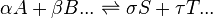$\alpha A +\beta B ... \rightleftharpoons \sigma S+\tau T ...$

the equilibrium constant can be defined by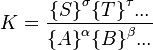$K=\frac{{\{S\}} ^\sigma {\{T\}}^\tau ... } {{\{A\}}^\alpha {\{B\}}^\beta ...}$

where {A} is the activity of the chemical species A, etc. (activity is a dimensionless quantity). It is conventional to put the activities of the products in the numerator and those of the reactants in the denominator.

For equilibria in solution, activity is the product of concentration and activity coefficient. Most chemists determine equilibrium constants in a solution with a high ionic strength. In high strength solutions, the quotient of activity coefficients changes very little. So, the equilibrium constant is defined as a concentration quotient: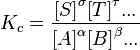$K_c=\frac{{[S]} ^\sigma {[T]}^\tau ... } {{[A]}^\alpha {[B]}^\beta ...}$

However, the value of Kc will depend on the ionic strength. (The square brackets mean the concentration of A, B and so on.)

This is a simple idea. In an equilibrium, atoms can combine or break apart because the reaction can work in both directions. For the reaction to work, all of the parts must be present to combine. This is more likely to happen if the reactants have a higher concentration. So, the concentrations of all the necessary pieces are multiplied together to get the probability that they will be in the same place for the reaction. (If the reaction requires two molecules of a particular compound, then the concentration of that compound is squared.) Going the other way, all of the concentrations of those necessary pieces are multiplied together to get the probability that they will be in the same place to react in the opposite direction. The ratio between those two numbers represents how popular each side of the reaction will be when equilibrium is reached. An equilibrium constant of 1 means that both sides are equally popular. Chemists perform experiments to measure the equilibrium constant of various reactions.

There is a relation between Gibbs free energy (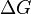$\Delta G$) and Equilibrium constant which is,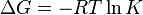$\Delta G = -RT \ln K$

Here,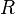$R$ is the universal gas constant and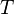$T$ is the temperature.

## Relation between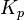$K_p$ and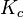$K_c$

From ideal gas law, we know that, ,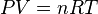$PV = nRT\,$

Or,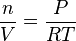$\frac{n}{V} = \frac{P}{RT}$

So, concentration (as, concentration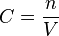$C = \frac{n}{V}$),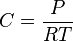$C = \frac{P}{RT}$

Here,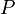$P$ is pressure,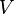$V$ is volume,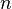$n$ is the number of moles of gas,$R$ is the universal gas constant and$T$ is the temperature. So,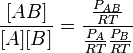$\frac{[AB]}{[A][B]} = \frac{\frac{P_{AB}}{RT}}{{\frac{P_A}{RT}}{\frac{P_B}{RT}}}$

Or,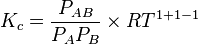$K_c = \frac{P_{AB}}{{P_A}{P_B}}\times {RT}^{1 +1 -1}$

Here,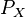$P_{X}$ is the partial pressure of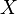$X$.

If,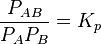$\frac{P_{AB}}{{P_A}{P_B}} = K_p$

Then,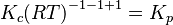$K_c {(RT)}^{-1 -1 +1} = K_p$

Here,$K_p$ is the equilibrium constant in terms of partial pressure.

In this same process,$\alpha A +\beta B ... \rightleftharpoons \sigma S+\tau T ...$

For the reaction above,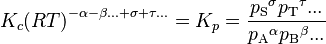$K_c {(RT)}^{-\alpha -\beta ... +\sigma +\tau ...} = K_p = \frac{{p_\mathrm{S}}^\sigma {p_\mathrm{T}}^\tau ...} {{p_\mathrm{A}}^\alpha {p_\mathrm{B}}^\beta ...}$

So, the relation between$K_c$ and$K_p$ is,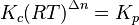$K_c {(RT)}^{\Delta n} = K_p$

Here,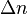$\Delta n$ the number of moles of gas on the product side minus the number of moles of gas on the reactant side in the balanced reaction

## Images for kidsEquilibrium constant Facts for Kids. Kiddle Encyclopedia.